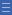## Featured resource

Home > Topdrawer > Fractions > Good teaching > Adding and subtracting

## Default object view. Click to create a custom template, Node ID: 12056, Object ID: 19882

Students should have opportunities to continue developing their fraction sense as they begin adding and subtracting fractions. Moving too quickly into standard procedures can inhibit understanding of strategies.

Students begin by modelling addition and subtraction of fractions with the same denominator in years 4 and 5, then progress to working with fractions that have related denominators in year 6. This forms the foundation for being able to find common denominators for any fractions in year 7.

There are various approaches to take.

• Count by fractions and 'jump' forwards and backwards along a number line.
• Apply a sense of the size of fractions (e.g. Predict whether $$\frac{3}{4}$$ + $$\frac{1}{8}$$ will be less than, equal to, or greater than 1. Explain how you know.)
• Use familiar models such as area diagrams (circles and squares) or a fraction wall.
• Use grids and arrays to promote thinking about factors and multiples in the relationship between denominators.

Student learning can be further supported by attention to:

• expressing fractional numbers greater than one as improper fractions and mixed numbers
• working on problems that provide a context to make sense of adding and subtracting fractions
• further development of strategies for recognising and creating equivalent fractions.

Yes

Yes

Name Class SectionSame denominator Folder 17Related denominators Folder 17Year 4: Count by quarters halves and thirds, including with mixed numerals. Locate and represent these fractions on a number line Infobox 3Year 5: Investigate strategies to solve problems involving addition and subtraction of fractions with the same denominator Infobox 3Year 6: Solve problems involving addition and subtraction of fractions with the same or related denominators Infobox 3Source Infobox 3